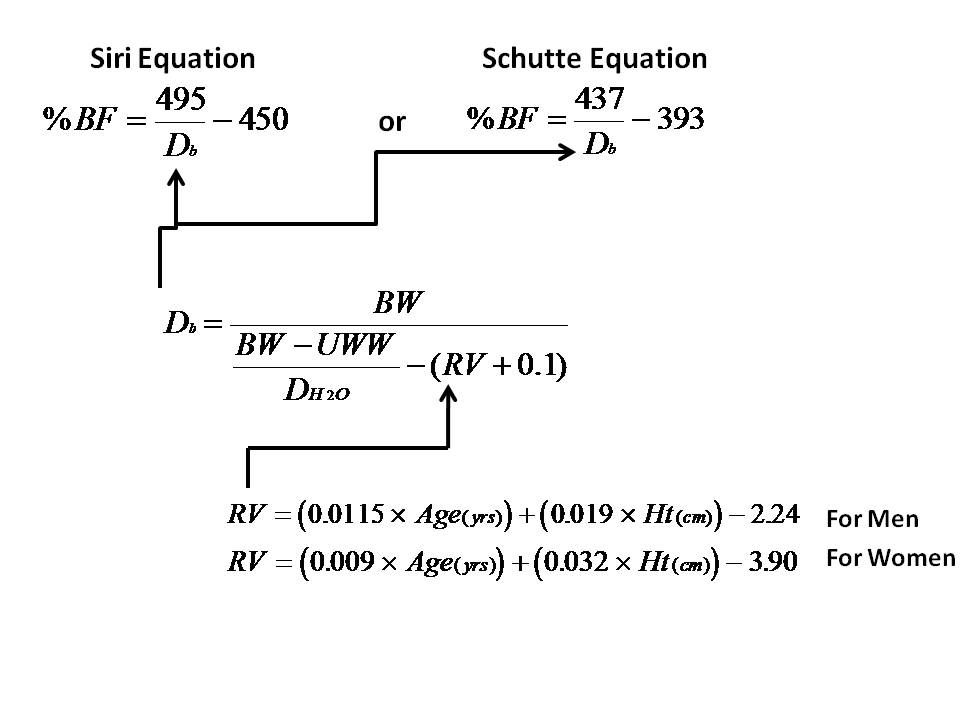# 4.1: Body Density

$$\newcommand{\vecs}{\overset { \rightharpoonup} {\mathbf{#1}} }$$ $$\newcommand{\vecd}{\overset{-\!-\!\rightharpoonup}{\vphantom{a}\smash {#1}}}$$$$\newcommand{\id}{\mathrm{id}}$$ $$\newcommand{\Span}{\mathrm{span}}$$ $$\newcommand{\kernel}{\mathrm{null}\,}$$ $$\newcommand{\range}{\mathrm{range}\,}$$ $$\newcommand{\RealPart}{\mathrm{Re}}$$ $$\newcommand{\ImaginaryPart}{\mathrm{Im}}$$ $$\newcommand{\Argument}{\mathrm{Arg}}$$ $$\newcommand{\norm}{\| #1 \|}$$ $$\newcommand{\inner}{\langle #1, #2 \rangle}$$ $$\newcommand{\Span}{\mathrm{span}}$$ $$\newcommand{\id}{\mathrm{id}}$$ $$\newcommand{\Span}{\mathrm{span}}$$ $$\newcommand{\kernel}{\mathrm{null}\,}$$ $$\newcommand{\range}{\mathrm{range}\,}$$ $$\newcommand{\RealPart}{\mathrm{Re}}$$ $$\newcommand{\ImaginaryPart}{\mathrm{Im}}$$ $$\newcommand{\Argument}{\mathrm{Arg}}$$ $$\newcommand{\norm}{\| #1 \|}$$ $$\newcommand{\inner}{\langle #1, #2 \rangle}$$ $$\newcommand{\Span}{\mathrm{span}}$$$$\newcommand{\AA}{\unicode[.8,0]{x212B}}$$

## Body Fat Percentage from Body Density

The pitfalls of the previously discussed skinfold method and the BMI can be somewhat avoided by actually measuring body density for use in empirical formulas that approximate body fat percentage:Formulas used in calculating residual lung volume, body density, and body fat percentage. Image Credit: Measure Body Fat Via Under Water Weighing by via Instructables

Your lab for this unit might involve some of these formulas and if you are curious you can read more about those formulas, play with a simulation of hydrostatic weighing, check out a website that does the calculations for you, and see that different formulas have been developed for different population sets in an effort to increase accuracy..

## Body Density

In order to understand density and how it might be measured, we need to know that volume (V) is the amount of space taken up by an object and mass (m) is a measure of the amount of matter contained in the object. For the body and other everyday objects matter refers to the atoms that make up the object and the number and type of atoms determines the mass. Later we will see that mass plays an important role in determining the weight and motion of objects. The SI units for volume and mass are cubic meters (m3) and kilograms (kg). MassDensity (ρ), which we usually shorten to just density, for any object is defined as its mass divided by its volume. The same mass of different materials will have different volume, and thus different densities. For example 1 kg of foam takes up much more space than 1 kg of steel (in fact, about 80 times more). This giant table of material densities is a useful reference (click the kg/m3 button to see the values in SI units).

Sometimes weight density is used instead of mass density, in which case weight (pull of gravity on an object) rather than mass is divided by volume. The following chapters will explain how we measure the volume, weight, and mass of a body in order to calculate body density for use in determining body composition.

This page titled 4.1: Body Density is shared under a CC BY-NC-SA 4.0 license and was authored, remixed, and/or curated by Lawrence Davis (OpenOregon) via source content that was edited to the style and standards of the LibreTexts platform; a detailed edit history is available upon request.